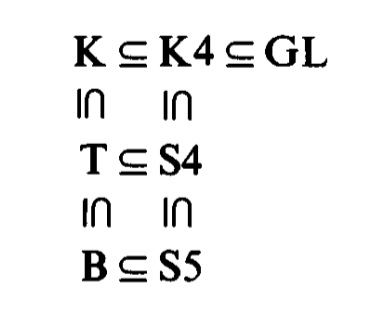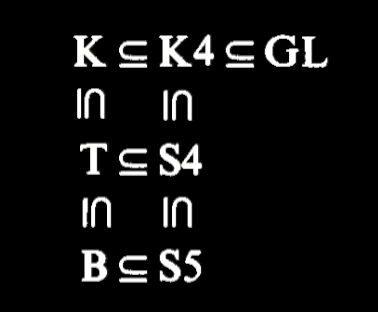# Normal system of provability logic

Between the modal systems of provability, the normal systems distinguish themselves by exhibiting nice properties that make them useful to reason.

A normal system of provability is defined as satisfying the following conditions:

1. Has necessitation as a rule of inference. That is, if $$L\vdash A$$ then $$L\vdash \square A$$.

2. Has modus ponens as a rule of inference: if $$L\vdash A\rightarrow B$$ and $$L\vdash A$$ then $$L\vdash B$$.

3. Proves all tautologies of propositional logic.

4. Proves all the distributive axioms of the form $$\square(A\rightarrow B)\rightarrow (\square A \rightarrow \square B)$$.

5. It is closed under substitution. That is, if $$L\vdash F(p)$$ then $$L\vdash F(H)$$ for every modal sentence $$H$$.

The simplest normal system, which only has as axioms the tautologies of propositional logic and the distributive axioms, it is known as the K system.

## Normality

The good properties of normal systems are collectively called normality.

Some theorems of normality are:

• $$L\vdash \square(A_1\wedge ... \wedge A_n)\leftrightarrow (\square A_1 \wedge ... \wedge \square A_n)$$

• Suppose $$L\vdash A\rightarrow B$$. Then $$L\vdash \square A \rightarrow \square B$$ and $$L\vdash \diamond A \rightarrow \diamond B$$.

• $$L\vdash \diamond A \wedge \square B \rightarrow \diamond (A\wedge B)$$

## First substitution theorem

Normal systems also satisfy the first substitution theorem.

(First substitution theorem) Suppose $$L\vdash A\leftrightarrow B$$, and $$F(p)$$ is a formula in which the sentence letter $$p$$ appears. Then $$L\vdash F(A)\leftrightarrow F(B)$$.

## The hierarchy of normal systems

The most studied normal systems can be ordered by extensionality:Those systems are:

• The system K

• The system K4

• The system GL

• The system T

• The system S4

• The system B

• The system S5

Parents: